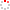NSSC OpenIR  > 空间科学部
 Modelling energetic particles by a relativistic kappa-loss-cone distribution function in plasmas Xiao, F. L.; 北京8701信箱 Department 空间天气学国家重点实验室 Source Publication Plasma Physics and Controlled Fusion2006 Volume 48Issue:2Pages:203-213 ISSN 0741-3335 Language 英语 Keyword Dispersion Function Electrons Instability Waves Magnetosphere Equilibrium Spectra Ion Abstract Energetic particles found in planetary magnetospheres and other plasmas, where mirror geometries occur, often exhibit two typical characteristics: a pronounced high energy tail and an anisotropy. A relativistic kappa-loss-cone (KLC) distribution function f(kappa L) is initially developed which incorporates features of the well-known kappa type and loss-cone type, i.e. the anisotropy behaves as a loss-cone distribution; the energy satisfies proportional to [1/nu(2)]((kappa,1)) for a relatively large velocity nu as a kappa distribution f(kappa) does and spreads proportional to [1/p](kappa+1) at the relativistic energies (where kappa and p are the energy spectral index and the particle momentum, respectively). This indicates that the new distribution f(kappa L) obeys the power-law not only at the lower energies but also at the relativistic energies since the relativistic energy proportional to p. Numerical calculations are performed for a direct comparison between the new KLC distribution and the current kappa distribution, respectively. It is found that the regular kappa distribution generally decreases faster than the KLC distribution with the kinetic energy E-k especially when theta(2) increases (where theta(2) is the energy weight parameter), e-g. f(kappa)/f(kappa L) <= 10(-2) for E-k >= 2.0 MeV and theta(2) >= 0.25. However, no big difference occurs between both distributions through energies up to similar to 500 keV for theta(2) <= 0.025. Furthermore, the regular kappa distribution containing either the temperature anisotropy or both the loss cone and temperature anisotropy is quite different from the KLC distribution. The new KLC distribution may be applicable to the outer radiation belts of the Earth, the inner Jovian magnetosphere and other plasmas (including the laboratory machine) where relativistic particles are present. Indexed By SCI Funding Project 中国科学院空间科学与应用研究中心 Document Type 期刊论文 Identifier http://ir.nssc.ac.cn/handle/122/1055 Collection 空间科学部 Corresponding Author 北京8701信箱 Recommended CitationGB/T 7714 Xiao, F. L.,北京8701信箱. Modelling energetic particles by a relativistic kappa-loss-cone distribution function in plasmas[J]. Plasma Physics and Controlled Fusion,2006,48(2):203-213. APA Xiao, F. L.,&北京8701信箱.(2006).Modelling energetic particles by a relativistic kappa-loss-cone distribution function in plasmas.Plasma Physics and Controlled Fusion,48(2),203-213. MLA Xiao, F. L.,et al."Modelling energetic particles by a relativistic kappa-loss-cone distribution function in plasmas".Plasma Physics and Controlled Fusion 48.2(2006):203-213.